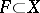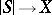# Simplicial space

(diff) ← Older revision | Latest revision (diff) | Newer revision → (diff)
A topological spaceequipped with a covering by topological simplices (called a triangulation) such that the faces of every simplex belong to the triangulation, the intersection of any two simplices is a face of each (possibly empty), and a subsetis closed if and only if its intersection with every simplex is closed. Every simplicial space is a cellular space. The specification of a triangulation is equivalent to the specification of a homeomorphism, whereis the geometric realization of some simplicial complex. Simplicial spaces are also called simplicial complexes or simplicial decompositions. Simplicial spaces are the objects of a category whose morphismsare mappings such that every simplex of the triangulation ofis mapped linearly onto some simplex of the triangulation of. The morphisms are also called simplicial mappings.